# Examples of Triangulation

## Delauney triangulation of many points in xy plane

25 points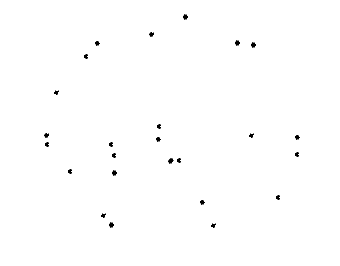compute convex hull of points: Point3d[] const hull: Point3d[] = [];
const interior: Point3d[] = [];
Point3dArray.computeConvexHullXY(points, hull, interior, true);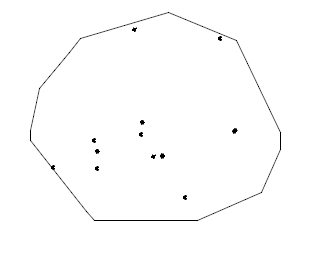One step points: Point3d[] to Polyface const polyface = PolyfaceBuilder.pointsToTriangulatedPolyface(points);
IndexedPolyface with all the points triangulated.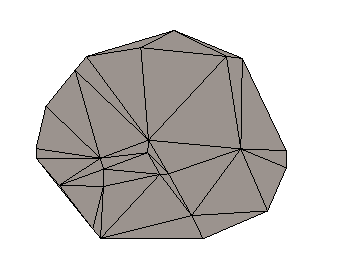Unit Test

• source: core\geometry\src\test\topology\InsertAndRetriangulateContext.test.ts
• test name: "TriangulateInHull"
• output: core\geometry\src\test\output\InsertAndRetriangulateContext\TriangulateInHull.imjs

## Triangulate points "between linestrings"

4 and 6 sided polygons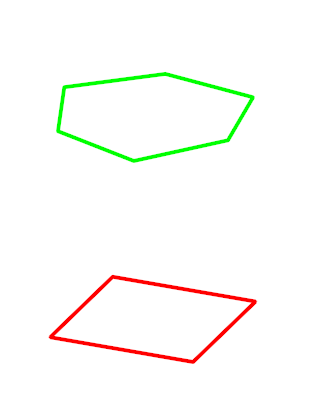Same polygons displayed with <br>handles to indicate the two linestrings have edge subdivision mismatch <br> in addition to the different cornering angles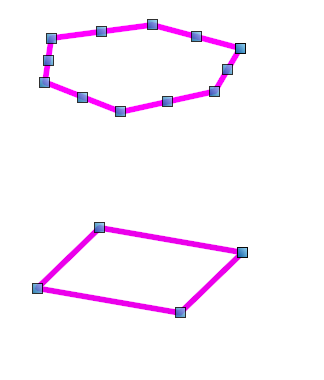triangles constructed "between" the polygons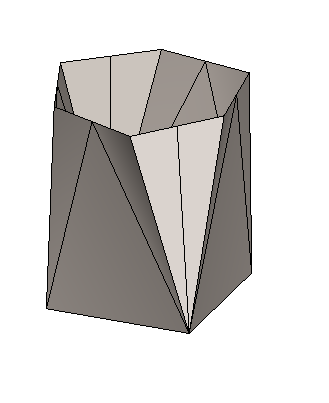Same polygons, another mix of points along edges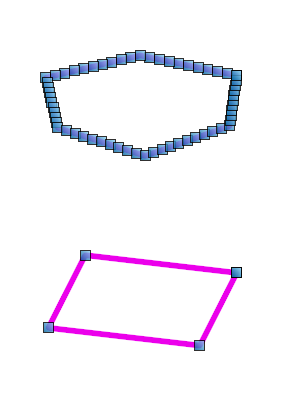triangles constructed "between" the polygons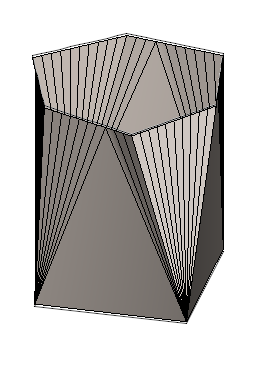Unit Test

• source: core\geometry\src\test\Polyface\GreedyTriangulationBetweenLineStrings.test.ts
• test set: describe("GreedyTriangulationBetweenLineStrings"
• test name: quadStar